# Integral Calculator with Steps

2.4
165 reviews
50K+Everyone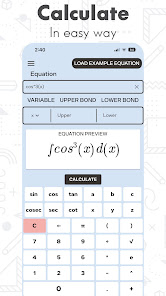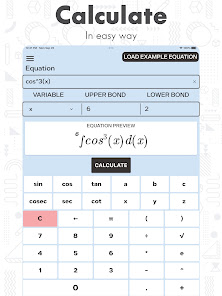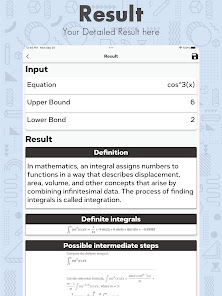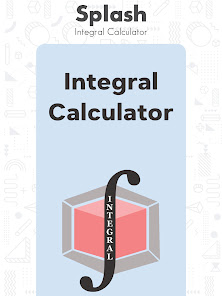Integral Calculator integration Solver with Steps is a smart and very easy to use calculator to measure integral equation problems and provide you with an accurate solution of Integral Equations.

It is hard for students to clearly understand and Measure Integration Questions due to lack of math knowledge. If you are weak to solve integral equation problems? Don’t worry, because this calculator is a complete math tutor for students of all classes and levels. Purpose of this Integration Calculator is to provide you with the easiest ways to Solve Integration Problems with Steps.

Integral is a commonly used function of math in derivative, while differentiating and estimating under curve area of any function graph, regulates a given function of Integration. This calculator gives you simple ways to solve integration problems step by step. Use this Integrals Calculator and make your math life easy by solving Integral Equation Problems.

Integration is used to calculate length, field, center point and functions for Integral Equation Solutions. This calculator is a gift for teachers and students of calculus because it is a quick Integration Solver with an easy to use Integral Calculator. It is easy to choose the type of Integrals, insert your question and get a quick answer by using Integration Calculator with Steps.

Features of Integral Calculator Integration Solver with Steps
There is a wide range of integrals and this calculator contains most used types of integration:
- Definite integrals
- Indefinite integrals
- Very easy to use calculator.
- Small size app to measure integral solutions.
- All functions of integrations at one place.
- Cool interface to easily use an Integral Calculator.
- Save time to solve integrations.
- Detailed report of integral equations solutions.
- Suitable for all math students.
- Integral Calculator With Steps.
- All integration formulas and functions.
- Complete calculator for math to Solve Integrals.

It is an Online Integral Calculator which helps you to Solve Integration Problems. No need to worry about which type of integral equation is in front of you to solve, when you have this smart app of Integration Solver Step by Step.

How to Measure Integrations and Solve Integrals Equations
Integral Calculator Integration Solver with Steps is an online calculator which comprises all functionalities and tools to measure and solve all infinite integrals, indefinite and definite integral of all available functions of integration. It takes only micro seconds to measure integration and provide you with a detailed solution of integration problem with steps. So that, you’ll be able to understand each step to Measure Integral Solution.

Don’t forget to apply boundaries to solve definite integrals, if you are using Integral Calculator to solve indefinite and definite multiple variables.

If you are looking for a good integrals solver but unable to find a real working integration solving app? We suggest using this integration calculator to get accurate results of integrals with steps to solve integration problems.

This easy to use Integration Calculator is a very good app to solve math questions of integrals. It allows you to easily calculate any integral online and verify its solution by measuring step by step results of Integral Calculator Solver.

Let’s make your life easy with Integral Calculator Integration Solver with Steps and start using integral equation solver with this Integration Solving App.
Updated on
Sep 29, 2022

## Data safety

Safety starts with understanding how developers collect and share your data. Data privacy and security practices may vary based on your use, region, and age. The developer provided this information and may update it over time.This app may share these data types with third parties
LocationNo data collected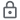Data is encrypted in transit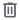You can request that data be deleted
2.4
165 reviews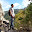John Eberlein
November 27, 2021
It seems like it would be a good app with the UI and design. But it didn't solve the expression I typed in correctly. I tried it four times thinking that I had a syntax error, and I added more parentheses to make sure. I wouldn't trust it for doing math, which is kinda important in a calculator app. I was trying to integrate: 1/(sqrt(1+x^2)) It would only give the answer for 1/(sqrt(x^2)) which is not at all the same thing.
7 people found this review helpful
Did you find this helpful?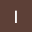Ibraheem Maqbool
August 17, 2022
Being a Mathematician. This app really helps me out solving problems. It's crucial for solving such time taking calculations. Best part of this app is the integration solver .Keep it up. ❤️
Did you find this helpful?
Bazzigate corp
August 18, 2022
Dear User, we are happy to know that you found our app useful. Please help us by spreading the word amongst your friends. If you have any feedback or suggestions, please write to us at bazzigatecorp@gmail.com. We would love to hear from you!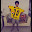Shail Shah
September 26, 2022
Just Amazing ! Tomorrow is my BE Sem 3 Mathematics exam and this is helping me so much !
Did you find this helpful?
Bazzigate corp
September 28, 2022
Wow, we are glad to know that. Thanks for your review! Please recommend our app to your friends, and don’t hesitate to shoot us a note at bazzigatecorp@gmail.com if you have any questions.